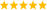# LabVIEW

cancel
Showing results for
Did you mean:

Solved!
Go to solution

## Convert 1D Array To Numeric Then Back To Array

Hello, I have a 1D array that contains the following:

Element 0 = MSByte of chip register (U8)

Element 1 = LSByte of chip register (U8)

What I want to do is take this 1D array convert it to numeric representation like the following:

Example if:

Element 0 = 1A hex

Element 1 = 44 hex

Conversion = MSM | LSB = 1A44 hex = 6724 dec

Then I want to AND this value with another value (0248 hex):

1A44 hex  ANDed with 0248 hex = 0240 hex

Then I want to take 0240 hex and split it back up into a 1D array like the following:

Element 0 = 02

Element 2 = 40

Is there an efficient way to take a 1D array, concatenate the arrays inputs, perform an AND function with another number, and then take the ANDed result and convert this value back to a 1D array with an MSByte (Element 0) and an LSByte (Element 1)?

Thanks!

Message 1 of 3
(1,381 Views)
Solution
Accepted by topic author hobby1

## Re: Convert 1D Array To Numeric Then Back To Array

Why don't you just AND it with an U8 array of x02,x48. No conversion or typecasting needed.(If you really need the intermediary U16 values too, just typecast the array to a scalar U16, etc.)LabVIEW Champion. It all comes together inGCentralWhat does "Engineering Redefined" mean??
Message 2 of 3
(1,374 Views)

## Re: Convert 1D Array To Numeric Then Back To Array

Thanks altenback.  I knew there was an efficient way to do this.

Message 3 of 3
(1,347 Views)